Polyabolo Puzzles
 All images © Abaroth. Permission is given to reproduce for non-profit purposes only. Home   Historic Sites   Models   Heraldry   Puzzles   Garden   Links

Tetrabolo Puzzles

These puzzles use the 14 tetraboloes. The pieces cover an area of 56 triangles, or 28 unit squares.

Geometric Forms

 Use all the pieces to make a symmetric pattern. Due to parity constraints, only patterns with bilateral symmetry are possible.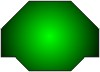Here is a page of these puzzles which you can print and solve: Tetrabolo Puzzles

Tri- and Tetrabolo Puzzles

These puzzles use the 4 triaboloes and 14 tetraboloes. The pieces cover an area of 68 triangles, or 34 unit squares.

Geometric Forms

 Use all the pieces to make a symmetric pattern. Here are examples for the different types of symmetry. A more difficult variation solves the overall pattern with two identical halves, each containing 2 triaboloes and 7 tetraboloes. Type of Symmetry Full Tetrarotary Double Bilateral Bilateral Double Diagonal Diagonal Birotary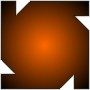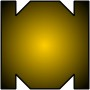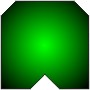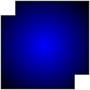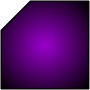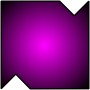Here is a page of these puzzles which you can print and solve: Tri- and Tetrabolo Puzzles

Pentabolo Puzzles

These puzzles use the 30 pentaboloes. The pieces cover an area of 150 triangles, or 75 unit squares.

Geometric Forms

 Use all the pieces to make a symmetric pattern. Here are examples for the different types of symmetry. Type of Symmetry Full Tetrarotary Double Bilateral Bilateral Double Diagonal Diagonal Birotary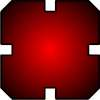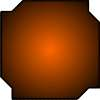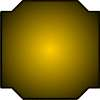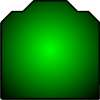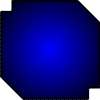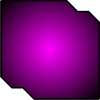Here are two pages of these puzzles which you can print and solve: Pentabolo Puzzles, Pentabolo Puzzles 2

Similar Geometric Pairs

 Use all the pieces to make a two copies of a symmetric pattern. The smaller copy using 10 pieces, and the larger using 20. The larger pattern will be rotated at 45 degrees to the smaller, so the orthogonal symmetries of one become the diagonals in the other. Here are examples for the different types of symmetry. Type of Symmetry Full Tetrarotary Double Reflective Reflective Birotary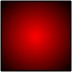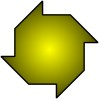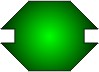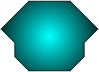Here is a page of these puzzles which you can print and solve: Pentabolo Pairs

Baiocchi Figures

 A Baiocchi Figure is one with maximal symmetry for the set of polyforms. You can find more about Baiocchi Figures at George Sicherman's Polyform Curiosities website. Here are the minimal solutions for the tetra- and pentaboloes along with the unsolvable pieces. Most of these were originally found by George.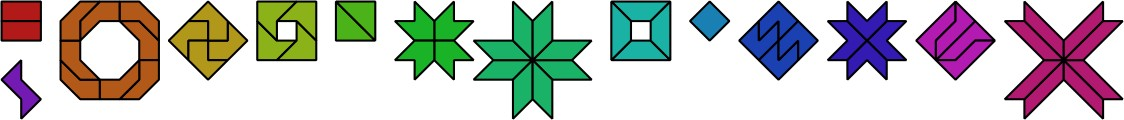Home   Historic Sites   Models   Heraldry   Puzzles   Garden   Links

Contact me with suggestions, comments or questions.